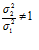Enjoy learning Statistics Online! Please be sure to share and subscribe to our YouTube channel.Course Documents

Chapter 1 - Intro

Chapter 2 - Methods for Describing Sets of Data

Chapter 3 - Probability

Chapter 4 - Discrete Random Variables

Chapter 5 - Normal Random Variables

Chapter 6 - Sampling Distributions

Chapter 7 - Confidence Intervals

Chapter 8 - Tests of Hypothesis: One Sample

Chapter 9 - Confidence Intervals and Hypothesis Tests: Two SamplesSample Exam I: Chapters 1 & 2

Sample Exam II: Chapters 3 & 4

Sample Exam III: Chapters 5 & 6

Sample Exam IV: Chapters 7 & 8

### Problem 2

9.10 Using the f-table to Find Critical Values

Use the f-table to find the critical value for each of the given scenario:

Sample 1: variance = 232, n1 = 30  Sample2: variance = 387, n2 = 46

Claim:Significance Level: 0.05

« back